# Samacheer Kalvi Books: Tamilnadu State Board Text Books Solutions

## Samacheer Kalvi 12th Computer Science Chapter 14 Importing C++ Programs in Python Notes PDF Download: Tamil Nadu STD 12th Computer Science Chapter 14 Importing C++ Programs in Python NotesSamacheer Kalvi 12th Computer Science Chapter 14 Importing C++ Programs in Python Notes PDF Download: Tamil Nadu STD 12th Computer Science Chapter 14 Importing C++ Programs in Python Notes

## Samacheer Kalvi 12th Computer Science Chapter 14 Importing C++ Programs in Python Notes PDF Download

We bring to you specially curated Samacheer Kalvi 12th Computer Science Chapter 14 Importing C++ Programs in Python Notes PDF which have been prepared by our subject experts after carefully following the trend of the exam in the last few years. The notes will not only serve for revision purposes, but also will have several cuts and easy methods to go about a difficult problem.

 Board Tamilnadu Board Study Material Notes Class Samacheer Kalvi 12th Computer Science Subject 12th Computer Science Chapter Chapter 14 Importing C++ Programs in Python Format PDF Provider Samacheer Kalvi Books

## How to Download Samacheer Kalvi 12th Computer Science Chapter 14 Importing C++ Programs in Python Notes PDFs?

2. Click on the Samacheer Kalvi 12th Computer Science Notes PDF.
3. Look for your preferred subject.
4. Now download the Samacheer Kalvi 12th Computer Science Chapter 14 Importing C++ Programs in Python notes PDF.

## Download Samacheer Kalvi 12th Computer Science Chapter 14 Importing C++ Programs in Python Chapterwise Notes PDF

PART – I

Question 1.
Which of the following is not a scripting language?
(a) JavaScript
(b) PHP
(c) Perl
(d) HTML
(d) HTML

Question 2.
Importing C++ program in a Python program is called …………………………
(a) wrapping
(c) Interconnecting
(d) Parsing
(a) wrapping

Question 3.
The expansion of API is ……………………….
(a) Application Programming Interpreter
(b) Application Programming Interface
(c) Application Performing Interface
(b) Application Programming Interface

Question 4.
A framework for interfacing Python and C++ is ………………………….
(a) Ctypes
(b) SWIG
(c) Cython
(d) Boost
(d) Boost

Question 5.
Which of the following is a software design technique to split your code into separate parts?
(a) Object-oriented Programming
(b) Modular programming
(c) Low-Level Programming
(d) Procedure Oriented Programming
(b) Modular programming

Question 6.
The module which allows you to interface with the Windows operating system is ………………………….
(a) OS module
(b) sys module
(c) csv module
(d) getopt module
(a) OS module

Question 7.
getopt( ) will return an empty array if there is no error in splitting strings to …………………………..
(a) argv variable
(b) opt variable
(c) args variable
(d) ifile variable
(c) args variable

Question 8.
Identify the function call statement in the following snippet.
if_name ==’_main_’:
main(sys.argv[l:])
(a) main(sys.argvfl:])
(b) _name_
(c) _main_
(d) argv
(b) _name_

Question 9.
Which of the following can be used for processing text, numbers, images, and scientific data?
(a) HTML
(b) C
(c) C++
(d) PYTHON
(d) PYTHON

Question 10.
What does the name contain?
(a) C++ filename
(b) main( ) name
(c) python filename
(d) os module name
(c) python filename

PART – II

Question 1.
What is the theoretical difference between Scripting language and another programming language?

• The theoretical difference between the two is that scripting languages do not require the compilation step and are rather interpreted.
• For example, normally, a C++ program needs to be compiled before running whereas, a scripting language like JavaScript or Python needs not be compiled.
• A scripting language requires an interpreter while a programming language requires a compiler.

Question 2.
Differentiate compiler and interpreter?
Compiler:

1. Scans the entire program and translates it as a whole into machine code.
2. It takes a large amount of time to analyze the source code
3. The overall execution time is comparatively faster.
4. Programming language like C, C++ use compilers

Interpreter:

1. Translates program one statement at a time.
2. It takes less amount of time to analyze the source code.
3. The overall execution time is slower.
4. Programming languages like Python, Ruby use interpreters.

Question 3.
Write the expansion of

1. SWIG
2. MinGW

SWIG (Simplified Wrapper Interface Generator. Both C and C++)
MinGW (Minimalist GNU for Windows)

Question 4.
What is the use of modules?

• The use of modules to break down large programs into small manageable and organized files.
• Modules provide reusability of code.
• We can define our most used functions in a module and import them, instead of copying their definitions into different programs

Question 5.
What is the use of cd command? Give an example?
The syntax to change from c:\> to the folder where Python is located is
cd <absolute path>
where “cd” command refers to change directory and absolute path refers to the complete path where Python is installed.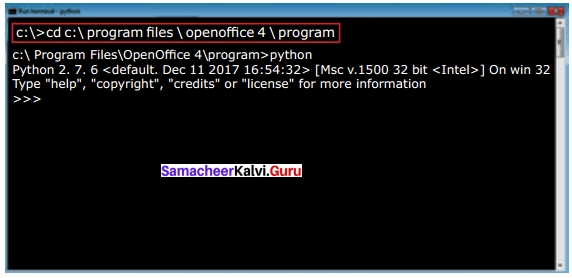In this Example to go to the folder where Python is located we should type the following command “cd C:\Program Files\OpenOffiice 4\Program”:

PART – III

Question 1.
Differentiate PYTHON and C++?
PYTHON:

1. Python is typically an “interpreted” language
2. Python is a dynamic- typed language
3. Data type is not required while declaring
4. It can act both as scripting and general-purpose language

C++:

1. C++ is typically a “compiled” language
2. C++ is compiled statically typed language
3. The data type is required while declaring a variable variable
4. It is a general-purpose language

Question 2.
What are the applications of a scripting language?
Applications of Scripting Languages

1. To automate certain tasks in a program
2. Extracting information from a data set
3. Less code-intensive as compared to traditional programming language
4. can bring new functions to applications and glue complex systems together

Question 3.
What is MinGW? What is its use?

• MinGW refers to a set of runtime header files, used in compiling and linking the code of C, C++, and FORTRAN to be run on the Windows Operating System. MinGw- W64 (a version of MinGW) is the best compiler for C++ on Windows.
• To compile and execute the C++ program, need ‘g++’ for Windows.
• MinGW allows to compile and execute C++ program dynamically through Python program using g++.

Question 4.
Identify the module, operator, definition name for the following
welcome.display( )
welcome – module name
– dot operator
display( ) – function name

Question 5.
What is sys.argv? What does it contain?

• sys.argv is the list of command-line arguments passed to the Python program.
• argv contains all the items that come along via the command-line input.
• It is basically an array holding the command-line arguments of the program.
• The first argument, sys.argv, isalways the name of the program as it was invoked, and sys.argv is the first argument we pass to the program

PART – IV

Question 1.
Write any 5 features of Python?

• Python uses Automatic Garbage Collection.
• Python is a dynamically typed language. Python runs through an interpreter.
• Python code tends to be 5 to 10 times shorter than that written in C++.
• In Python, there is no need to declare types explicitly whereas it should be done in C++
• In Python, a function may accept an argument of any type, and return multiple values without any kind of declaration beforehand.

Question 2.
Explain each word of the following command?
The syntax to execute the Python program is
Python <filename.py> -i <C++filename without cpp extension>
Where,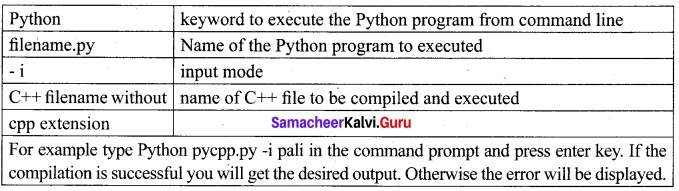Question 3.
What is the purpose of sys,os,getopt module in Python. Explain?
1. Python’s sys module
This module provides access to some variables used by the interpreter and to functions that interact strongly with the interpreter.

sys.argv:
sys.argv is the list of command-line arguments passed to the Python program, argv contains all the items that come along via the command-line input, it’s basically an array holding the command-line arguments of the program.

To use sys.argv, you will first have to import sys. The first argument, sys.argv, is always the name of the program as it was invoked, and sys.argv) 1] is the first argument you pass to the program (here it is the C++ file). For example

main(sys.argv[ 1 ]) Accepts the program file (Python program) and the input file (C++ file) as a list(array). argv contains the Python program which is need not to be passed because by default main contains source code reference and argv[l] contains the name of the C++ file which is to be processed.

2. Python’s OS Module
The OS module in Python provides a way of using operating system dependent functionality. The functions that the OS module allows you to interface with the Windows operating system where Python is running on.
os.system( ): Execute the C++ compiling command (a string contains Unix, C command which also supports C++ command) in the shell (Here it is Command Window). For Example to compile C++ program g++ compiler should be invoked. To do so the following command is used.
os.system (g++’ + <varciiable_namel> ‘-<mode>’+ <variable_name2>
where,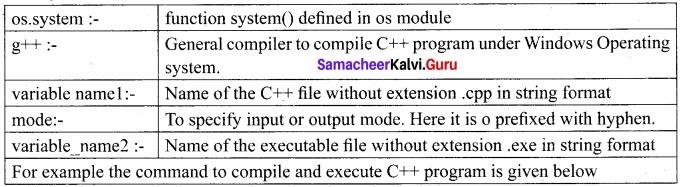os.system(‘g++ ‘+ cpp_file + ‘-o ‘+ exe_file) g++ compiler compiles the file_cpp_file and -o (output) send to exe file
Note
‘+’ in os.system( ) indicates that all strings are concatenated as a single string and send that as a List.

3. Python getopt module
The getopt module of Python helps you to parse (split) command-line options and arguments. This module provides two functions to enable command-line argument parsing, getopt.getopt method
This method parses command-line options and parameter list. Following is the syntax for this method
<opts>,<args>=getopt.getopt(argv, options, {long_options])
Here is the detail of the parameters –
argv – This is the argument list of values to be parsed (splited). In our program the complete command will be passed as a list.
options – This is string of option letters that the Python program recognize as, for input or for output, with options (like ‘i’ or ‘o’) that followed by a colon (:). Here colon is used to denote the mode.
long options – This parameter is passed with a list of strings. Argument of Long options should be followed by an equal sign (‘=’). In our program the C++ fde name will be passed as string and ‘i’ also will be passed along with to indicate it as the input file.
getopt( ) method returns value consisting of two elements. Each of these values are stored separately in two different list (arrays) opts and args. Opts contains list of splitted strings like mode, path and args contains any string if at all not splitted because of wrong path or mode, args will be an empty array if there is no error in splitting strings by getopt( ).
For example The Python code which is going to execute the C++ file p4 in command line will have the getopt( ) method like the following one. opts, args = getopt.getopt (argv, “i:”,[‘ifile=]) where opto contains [(‘-i’, ‘c:\ \pyprg\\p4’)]
-i: – option nothing but mode should be followed by:
‘c: \ \pyprg\\p4’ value nothing but the absolute path of C++ file.
In our examples since the entire command line commands are parsed and no leftover argument, the second argument args will be empty [ ]. If args is displayed using print( ) command it displays the output as [ ].
>>>print(args)
[ ]

Question 4.
Write the syntax for getopt( ) and explain its arguments and return values?
Python getopt module
The getopt module of Python helps you to parse (split) command-line options and arguments. This module provides two functions to enable command-line argument parsing, getopt.getopt method
This method parses command-line options and parameter list. Following is the syntax for this method –
<opts>,<args>=getopt.getopt(argx>, options, {.long_options])
Here is the detail of the parameters –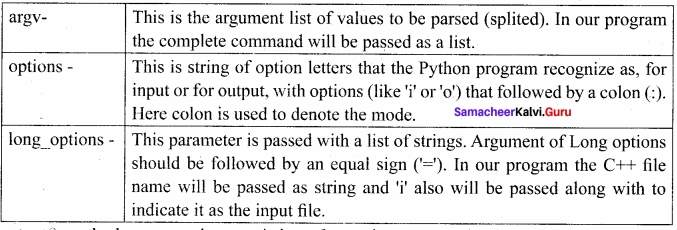getopt( ) method returns value consisting of two elements. Each of these values are storec separately in two different list (arrays) opts and args .Opts contains list of splitted strings like mode, path and args contains any string if at all not splitted because of wrong path or mode, args will be an empty array if there is no error in splitting strings by getopt( ).
For example The Python code which is going to execute the C++ file p4 in command line will have the getopt( ) method like the following one. opts, args = getopt.getopt (argv, “i:”,[‘ifile=’])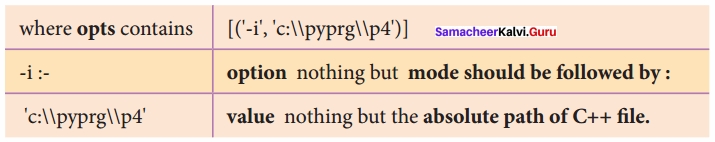In our examples since the entire command line commands are parsed and no leftover argument, the second argument args will be empty [ ]. If args is displayed using print( ) command it display the output as [ ].
>>>print(args)
[ ]
Some more command for wrapping C++ code
if_name_==’_main_’
main(sys.argv[1:])
_name_(A Special variable) in Python
Since there is no main() function in Python, when the command to run a Python program is given to the interpreter, the code that is at level 0 indentation is to be executed. However, before doing that, interpreter will define a few special variables. _name_ is one such special variable which by default stores the name of the file. If the source file is executed as the main program, the interpreter sets the _name_ variable to have a value as “_main_” name is a built-in variable which evaluates to the name of the current module. Thus it can be used to check whether the current script is being run on its own.
For example consider the following
if_name_ ==’_ main_’
main(sys.argv[1:])
if the command line Python program itself is going to execute first, then _main_ contains the name of that Python program and the Python special variable _name_ also contain the Python program name. If the condition is true it calls the main which is passed with C++ file as argument.

Question 5.
Write a Python program to execute the following C++ coding.
#include<iostream>
using namespace std;
int main()
{cout<<“WELCOME”;
return(0);
}
The above C++ program is saved in a file
welcome, cpp
#Now select File?Mop of Form-New in
Notepad and type the Python program as
main.py
# Program that compiles and executes a cpp file
# Python main.py -i welcome
import sys, os, getopt
def main(argv):
cpp_file =”
exe_file =”
opts, args = getopt.getopt(argv, “i:”,[‘ifile=’])
for o, a in opts:
if o in (“-i”, “–ifile”):
cpp_file = a + ‘.cpp’
exe_file = a + ‘.exe’
run(cpp_file, exe_file)
def run(cpp_file, exe_file):
print(” Compiling” + cpp_file)
os.system(‘g++ ‘ + cpp_file +’ -0′ + exe_ file)
print(” Running” + exe_file)
print(” — —- ——–“)
print
os. sy stem(exe_f ile)
print
if _name_ — _main_’:
main(sys.argv[1:])

Practice Programs

Question 1.
Write a C++ program to create a class called Student with the following details?
Protected member
Rno integer
Public members
void Readno(int); to accept roll number and assign to Rno
void Writeno( ); To display Rno.
The class Test is derived Publically from the Student class contains the following details
Protected member
Mark1 float
Mark2 float
Public members
void Readmark(float, float); To accept mark1 and mark2
void Writemark( ); To display the marks
Create a class called Sports with the following detail
Protected members
score integer
Public members
void Readscore(int); To accept the score
void Writescore( ); To display the score
The class Result is derived Publically from Test and Sports class contains the following – details
Private member
Total float
Public member
void display( ) assign the sum of mark1, mark2, score in total
invokeWriteno( ), Writemark( ) and Writescore( ). Display the total also.
Save the C++ program in a file called hybrid. Write a python program to execute the hybrid.cpp
In Notepad, type the C++ program
#include<iostream>
using namespace std;
class student
{
protected:
int mo;
public:
{
mo = rollno;
}
void writeno( )
{
cout<< “\n Roll no:” <<rno;
}};
class test: public student
{
protected:
float mark1,mark2;
public:
{
mark1 = m1;
mark2 = m2;
}
void writemark( )
{
cout<< “\n mark1 ” << mark1;
cout<< “\n mark2 ” <<mark2;
}};
class sports
{
protected:
int score;
public:
{
score = s;
}
void writescore( )
{
cout<< “SCORE : ” <<score;
}};
class result: public test, public sports
{
private: float total; public:
void display( )
{
total = mark1 + mark2;
cout<< “TOTAL MARKS: ” <<total;
}};
int main( )
{
result r;
r.writeno( );
r.writemark( );
r.display( );
r.writescore( );
}
save this file as hybrid.cpp
Now type the python program in New Notepad file.
# python hybrid.py -i hybrid.cpp
import sys,os,getopt def main(argv): cpp Jile =” exe_file =”
opts, args = getopt.getopt(argv, “i:”,[‘ifile-])
for o, a in opts:
if o in(“-i”, “–file”):
cpp_file =”a+ ‘.cpp’
exe_file = a+ ‘.exe’
run(cpp_file, exefile)
def run(cpp_file, exe_file):
print(“Compiling” + cpp_file)
os.system(‘g++ ‘+ cpp_file + ‘-o ‘+ exe_file)
print(“Running” + exefile)
print(“———————“)
print
os.system(excfile)
print
if name ==’ main
main(sys.argv[1:])
Output:
Rollno : 5
Mark1 : 100
Mark2 : 100
TOTAL MARKS : 200
SCORE : 200

Question 2.
Write a C++ program to print boundary elements of a matrix and name the file as Border.cpp. Write a python program to execute the Border.cpp?
Select File → New in Notepad and type the C++ program.
#include<iostream>
#include<bits/stdc++.h>
using namespace std;
const int MAX =100;
void printBoundary(int a[][max], int m, int n)
{
for (int i=0; i < m; i++)
{
for(int j=0; j < n; j++)
{
if(i= =0 || j= =0 || i= =n-l ||j==n-l)
cout <<a[i][j]
<< ” else
cout <<“”
cout<<”
}
cout <<” \n”;
}}
int main( )
{
int a[MAX] = { {1,2,3,4}, {5,6,7,8}, {1,2,3,4}, {5,6,7,8}};
print Boundary(a,4,4);
return 0;
}
save it as Border.cpp
open a New notepad file and type the python program to execute border.cpp
#python border.py -i border.cpp
import sys,os,getopt
def main(argv):
cpp_file =”
exe_file =”
opts, args = getopt.getopt(argv, “i:”,[‘ifile=’])
for o, a in opts;
if o in(“-i”, “-ifile”):
cpp_file =a+ ‘.cpp’
exe_file = a+ ‘.exe’
run(cpp_file, exe_file)
def run(cpp_file, exe_file):
print(“Compiling” + cpp_file)
os.system(‘g++ ‘+ cpp_file + ‘-o ‘+ exe_file)
print(“Running” + exe_file)
print(“———————“)
print
os.system(exe_file)
print
if_name_==’_main_’:
main(sys.argv[1:])
Output: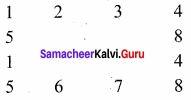### Samacheer Kalvi 12th Computer Science Importing C++ Programs in Python Additional Questions and Answers

Question 1.
In language data type or not required while declaring a variable.
a) C++
b) C
c) Java
d) Python
d) python

Question 2.
Find the correct statement.
(a) C++ is a dynamic typed language
(b) python is a dynamic typed language
(b) python is a dynamic typed language

Question 3.
_______________ is designed for integrating and communicating with other programming languages.
a) Modular language
b) Procedural language
c) Scripting language
d) Procedural language
c) Scripting language

Question 4.
Pick the odd one out Perl, Ruby, ASP, Tel, Java
Java

Question 5.
Read the statement given below and choose the correct option.
(I) All scripting languages are programming languages.
(II) A scripting language requires an interpreter
(III) Programming language requires a compiler
(a) (I) – False, (II), (III) – True
(b) (I), (II) – False
(c) All are true
(d) All are false
(c) All are true

Question 6.
__________ is an expansion of SWIG?
a) Simplified Wrapper Interface Generator
b) Software Wrapper Information Generator
c) Simplified Wrapper Interface Generator
d) System Wrapper Interface Generator
a) Simplified Wrapper Interface Generator

Question 7.
The process by which python periodically frees and reclaims block of memory that no longer are in use is called ……………………………..
Garbage Collection

Question 8.
………………………. is a python-like language for writing c extensions.
python

Question 9.
…………..is an array holding the command line arguments of the program
a) g++
b) argv
c) Opts
d) Getopt
b) argv

Question 10.
……………………. is the best compiler for C++ on windows.
(a) MinGW-w64
(b) MinGW-w63
(c) MinGW-w62
(d) MinGW-w60
(a) MinGW-w64

Question 11.
MinGW allows to compile and execute C++ program dynamically through python program using ……………………….
(a) C++
(b) g++
(c) a++
(d) x++
(b) g++

Question 12.
g++ is a program that calls GNU C compiler called …………………………….
(a) GCC
(b) CCC
(c) GGC
(d) GGG
(a) GCC

Question 13.
The command to change directory is …………………………….
(a) cc
(b) cd
(c) dc
(d) changed
(b) cd

Question 14.
………………………. keyword is used to execute the python program from the command line
Python

Question 15.
__________ module of python helps you to split command-line options and arguments.
a) OS
b) Getopt
c) Sys
d) All of these
b) Getopt

Question 16.
Which command is used to clear the screen?
(a) clear
(b) clean
(c) cls
(d) clrscr
(c) cls

Question 17.
……………………… refer to a file containing python statements and definitions.
Modules

Question 18.
pythons’ …………………………… provides access to the same variables and functions that interact with the interpreter.
sys module

Question 19.
Which is the list of command-line arguments passed to the python program.
(a) sys.ar
(b) sys.argv
(c) sys.sys
(d) sys.opt
(b) sys.argv

Question 20.
Match the following related to the OS module.
1. g++ – (i) Name of C++file
2. variable namel – (ii) General compiler
3. mode – (iii) input output mode
4. variable_name2 – (iv) Name of exe file
(a) 1-i, 2-ii, 3-iii, 4-iv
(b) 1-ii, 2-i, 3-iii, 4-iv
(c) 1-iv, 2-iii, 3-ii, 4-i
(d) 1-iv, 2-i, 3-iii, 4-ii
(b) 1-ii, 2-i, 3-iii, 4-iv

Question 21.
Which of the following is the special variable which by default stores the name of the file?
a) _name_
b) _init_
c) _del_
d) _def_
a) _name_

Question 22.
Which module helps to parse(split) command-line options and arguments?
(a) getopt
(b) argopt
(c) get
(d) putopt
(a) getopt

Question 23.
How many functions are there in getopt module to enable command-line argument parsing?
(a) 1
(b) 2
(c) 3
(d) 4
(b) 2

Question 24.
getopt mode is given by ………………………….
(a) ;
(b) =
(c) #
(d) :
(d) :

Question 25.
The argument of long options in the getopt method is followed by ………………………….
(a) ‘
(b) =
(c) #
(d) :
(b) =

Question 26.
When the command to run a python program is given to the interpreter, code at …………………………. indentation is executed.
(a) level 0
(b) level 1
(c) level 2
(d) level 3
(a) level 0

Question 27.
Which one is a special variable which by default stores the name of the file?
(a) main
(b) none
(c) _name_
(d) get
(c) _name_

Question 28.
The line number starts from …………………………… script.
python

Question 29.
The …………………………… definition invokes the g++ compiler and creates the exe file?

Question 30.
Which command of the os module executes the exe file?
(a) run
(b) system( )
(c) main
(d) name
(a) run

Question 31.
Which operator is used to access the functions of an imported value?
(a) +
(b) *
(c) .
(d) /
(c) .

Question 32.
………………………….. refers to a set of runtime header files used in compiling and linking the code of C, C++, Fortran to run on window os
(a) MaxGW
(b) CountGW
(c) MinGW
(d) AvgGW
(c) MinGW

PART – II

Question 1.
Differentiate statically typed language and dynamically typed language.

• A statically typed language like C++ requires the programmer to explicitly tell the computer what “data type” each data value is going to use.
• A dynamically typed language like Python doesn’t require the data type to be given explicitly for the data. Python manipulates the variable based on the type of value.

Question 2.
Write a note on Modular programming?
Modular programming is a software design technique to split your code into separate parts. These parts are called modules. The focus for this separation should have modules with no or just a few dependencies upon other modules.

PART – III

Question 1.
Name some commonly used Interfaces for importing C++ files on python?
Importing C++ Files in Python:
Importing a C++ program in a Python program is called wrapping up of C++ in Python. Wrapping or creating Python interfaces for C++ programs is done in many ways. The commonly used interfaces are

1. Python-C-API (API-Application Programming Interface for interfacing with C programs)
2. Ctypes (for interfacing with c programs)
3. SWIG (Simplified Wrapper Interface Generator. Both C and C++)
4. Cython (Cython is both a Python-like language for writing C-extensions)
5. Boost. Python (a framework for interfacing Python and C++)
6. MinGW (Minimalist GNU for Windows)

Question 2.
How will you import modules in python?
We can import the definitions inside a module to another module. We use the import keyword to do this. To import the module factorial we type the following in the Python prompt.
>>> import factorial
Using the module name we can access the functions defined inside the module. The dot(.)
the operator is used to accessing the functions. The syntax for accessing the functions from the module is
<module name>.<function name>
For example:
>>> factorial.fact(5)
120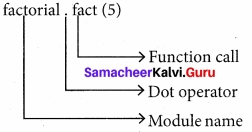Question 3.
Give the syntax for getopt module?
The syntax for this method
<opts> ,<args>=getopt.getopt(argv, options, [long_options])
Here is the detail of the parameters –Question 4.
Write the steps for executing the C++ program to check whether a given number is palindrome or not?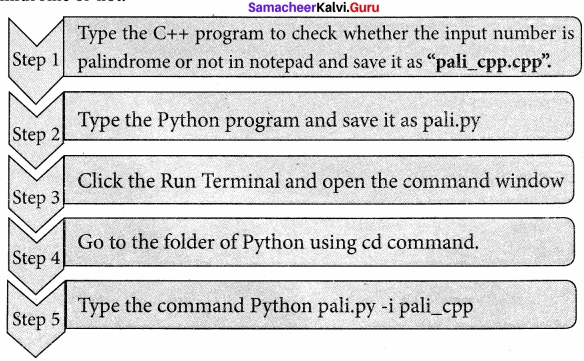PART – IV

Question 1.
Explain Pythons OS Module?
Python’s OS Module:
The OS module in Python provides a way of using operating system dependent functionality. The functions that the OS module allows you to interface with the Windows operating system where Python is running on.
os.system( ): Execute the C++ compiling command (a string contains Unix, C command which also supports C++ command) in the shell (Here it is Command Window). For Example, to – compile a C++ program g++ compiler should be invoked. To do so the following command is used.
os.system (g++’ + <varaiable_namel> ‘-<mode>’+ <variable_name2>
where,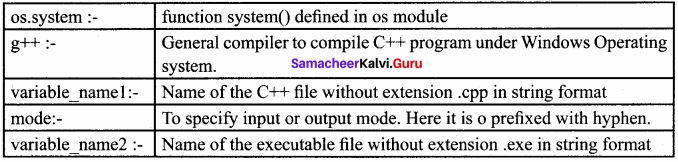For example the command to compile and execute C++ program is given below
os.system(’g++ ’+ cpp_file + ‘-o ‘+ exe_file) g++ compiler compiles the file cpp_file and -0(output) send to exe_file

Question 2.
Write a C++ program to enter any number and check whether the number is palindrome or not using a while loop.
/*. To check whether the number is palindrome or not using a while loop.*/
//Now select File->New in Notepad and type the C++ program
#include <iostream>
using namespace std;
int main()
{
int n, num, digit, rev = 0;
court<< “Enter a positive number: “;
cin>.num;
n = num;
while (num)
{ digit = num % 10;
rev = (rev * 10) + digit;
num = num / 10;}
cout<< ” The reverse of the number is: ”
<< rev <<endl;
if (n == rev)
cout<< ” The number is a palindrome”;
else
cout<< ” The number is not a
palindrome”;
return 0;
}
#Now select File→few in Notepad and type the Python program
# Save the File as pali.py Program that compiles and executes a .cpp file
# Python c:\pyprg\pali.py -i c:\pyprg\ pali_cpp
import sys, os, getopt
def main(argv):
cpp_file
exe_file = ”
opts, args = getopt.getopt(argv,
“i:”,[‘iffle=’])
for o, a in opts:
if o in (“-i”, “–ifile”):
cpp_file = a + ‘.cpp’
exe file = a + ‘.exe’
run(cpp_file, exe_file)
def run(cpp_file, exe_file):
print(” Compiling ” + cpp_file)
os.system(‘g++ ‘ + cpp_file + ‘ -o ‘ + exe_file)
print (“Running ” + exe_file)
print(“————– “)
print
os.system(exe_file)
print
if _name_== _main_ ‘: #program
starts executing from here
main(sys.argv[l:])
The output of the above program :
C:\Program Files\OpenOffice 4\
program>Python c:\pyprg\pali.py -i c:\ PyPrg\pali_cpp
Compiling c:\pyprg\pali_cpp.cpp
Running c:\pyprg\pali_cpp.exe
Enter a positive number : 56765
The reverse of the number is : 56765
The number is a palindrome

Question 3.
Write a C++ program to check whether the given number is palindrome or not. Write a program to execute it?
Write a C++ program to enter any number and check whether the number is palindrome or not using while loop?
//Now select File-> New in Notepad and type the C++ program
#include <iostream>
using namespace std;
int main( )
{
int a, i, j;
for(i=o; j<3;i++)
{
for(j=0;j<3;j++)
{count<<“enter the value for array [”<<i+1<<“]<<”<<“[“<<j+1<<“]”;
cin>>a[i][j];
}
}
system(“cls”); .
cout<<“\n \nOriginal Array\n”;
for(i=0; i<3; i++)
{
for(j=0; j<3; j++)
cout<<a[i] [j]
cout<<end1;
}
cout<<end1;
}
return 0;
}
// Save this file as trans_cpp.cpp
// Now select File → New in Notepad and type the Python program
#Save the File as transpose.py.Program that compiles and executes a .cpp file
#Python tanspose.py -i trans_cpp
import sys, os, getopt
def main(argv):
cpp_file =”
exefile =”
opts, args = getopt.getopt(argv, “i:”,[‘ifile=’])
for o, a in opts:
if o in (“-i”,–ifile”):
cpp_file =a+ ‘.cpp’
exe_file = a+ ‘.exe’
run(cpp_file, exe_file)
def run(cpp_file, exe file):
print(“Compiling” + cppfile)
os.system(‘g++’ + cpp_file + ‘-o ‘+ exe_file)
print(“Running” + exefile)
print(“———————-“)
print
os.system(exe_file)
print
if_name_==’_main_’:
main(sys.argv[1:])
Output of the above program
Original Array
1 2 3
4 5 6
7 8 9
The Transpose of Matrix
1 4 7
2 5 8
3 6 9

Question 4.
Write a C++ program to find the cube of a number. Write a python program to execute it?
/* Write a C++program using a user defined function to function cube of a number. */
//Now select File → New in Notepad and type the C++program
#include <iostream>
using namespace std;
//Function declaration
int cube(int num); int main( )
{
int num; int c;
cout<<“Enter any number: “<<end1;
cin>>num;
c = cube(num);
cout<<“Cube of’ <<num<<” is “<<c;
return 0;
}
//Function to find cube of any number
int cube(int num)
{
return (num * num * num);
}
//Save this file as cube_file.cpp
#Now select File → New in Notepad and type the Python program
#Save the File as fun.py
#Program that compiles and executes a .cpp file
#Python fun.py -i c:\pyprg\cuheJile.cpp
import sys, os, getopt
def main(argv):
cpp_Jile =”
exe_file =”
opts, args = getopt.getopt(argv, “i:”,[‘ifile-])
for o, a in opts:
if o in(“-i”, —ifile”):
cpp_file =a+ ‘.cpp’
exe_file = a+ ‘.exe’
run(cpp_file, exe_file)
def run(cpp_file, exe file):
print(“Compiling” + cppfile)
os.system(‘g++’+ cpp file + ‘-o ‘+ exe file)
print(“Running” + exe_file)
print(“——————–“)
print
os. sy stem(exe_file)
print
if_name_==’_main_’:
main(sys.argv[1:])
The output of the above program
Compiling c:\pyprg\cube_file.cpp
Running c:\pyprg\cube_file.exe
———————-
Enter any number:
5
Cube of 5 is 125

Question 5.
Write a C++ program to implement multilevel inheritance. Write a python program to execute it?
// C++program to implement Multilevel Inheritance
//Now select File → New in Notepad and type the C++program
#include <iostream>
using namespace std;
// base class
class Vehicle
{
public:
Vehicle( )
{
cout<< “This is a Vehicle” <<end1;
}
};
class threeWheeler: public Vehicle
{public:
threeWheeler( )
{
cout<<“Objects with 3 wheels are vehicles”<<end1;
}
};
// subclass derived from two base classes
class Auto: public three-wheeler
{
public:
Auto( )
{
cout<<“Auto has 3 Wheels”<<end1;
}
// main function
int main( )
{
//creating object of subclass will invoke the constructor of base classes
Auto obj;
return 0;
}
// Save this file as inheri_cpp.cpp
//Now select File —* New in Notepad and type the Python program
#Save the File as classpy.py
#Python classpy.py -i inheri_cpp command to execute C++ program
import sys, os, getopt
def main (argv):
cpp_file =”
exe file= 11
opts, args = getopt.getopt (argv, 11i: 11 ,[‘ifile=’])
for o, a in opts:
if o in (“-i”, —ifile”):
cpp_file =a+ ‘.cpp’
exe_file = a+ ‘.exe’
run (cpp file, exe_file)
def run(cpp_file, exe_file):
print (”Compiling 11 + cpp file)
os.system (‘g++’ + cpp_file +’ -o ‘+ exe_file)
print (“Running”+ exe file)
print(“———————“)
print
os.system (exefile) print
if_name_==’_main_’:
main (sys.argv[1:])
Output of the above program
Compiling c:\pyprg\class_file.cpp
Running c:\pyprg\class_file.exe
This is a Vehicle
Objects with 3 wheels are vehicles
Auto has 3 Wheels

## How to Prepare using Samacheer Kalvi 12th Computer Science Chapter 14 Importing C++ Programs in Python Notes PDF?

Students must prepare for the upcoming exams from Samacheer Kalvi 12th Computer Science Chapter 14 Importing C++ Programs in Python Notes PDF by following certain essential steps which are provided below.

• Use Samacheer Kalvi 12th Computer Science Chapter 14 Importing C++ Programs in Python notes by paying attention to facts and ideas.
• Pay attention to the important topics
• Refer TN Board books as well as the books recommended.
• Correctly follow the notes to reduce the number of questions being answered in the exam incorrectly
• Highlight and explain the concepts in details.

## Frequently Asked Questions on Samacheer Kalvi 12th Computer Science Chapter 14 Importing C++ Programs in Python Notes

#### How to use Samacheer Kalvi 12th Computer Science Chapter 14 Importing C++ Programs in Python Notes for preparation??

Read TN Board thoroughly, make separate notes for points you forget, formulae, reactions, diagrams. Highlight important points in the book itself and make use of the space provided in the margin to jot down other important points on the same topic from different sources.

#### How to make notes for Samacheer Kalvi 12th Computer Science Chapter 14 Importing C++ Programs in Python exam?

Read from hand-made notes prepared after understanding concepts, refrain from replicating from the textbook. Use highlighters for important points. Revise from these notes regularly and formulate your own tricks, shortcuts and mnemonics, mappings etc.
Share: